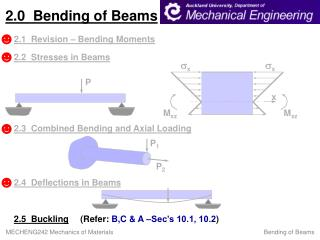DownloadDownload Presentation2 .0 Bending of Beams

# 2 .0 Bending of Beams

Télécharger la présentation## 2 .0 Bending of Beams

- - - - - - - - - - - - - - - - - - - - - - - - - - - E N D - - - - - - - - - - - - - - - - - - - - - - - - - - -
##### Presentation Transcript

1. sx sx P x Mxz Mxz P1 P2 2.0 Bending of Beams ☻ 2.1 Revision – Bending Moments ☻ 2.2 Stresses in Beams ☻ 2.3 Combined Bending and Axial Loading ☻ 2.4Deflections in Beams 2.5Buckling (Refer: B,C & A –Sec’s 10.1, 10.2)

2. Consider load, P, plotted against horizontal displacement, v. Point of Bifurcation P v 2.5Bars Under Axial Compression - Buckling (Refer: B, C & A–Sec 10.1, 10.2) Euler, Leonard (1741) For bars or columns under compressive loading, we may witness a sudden failure, known as buckling. This is a form of instability. P P Short, Thick Long, Slender

3. y v P P d y z L b x P x P Mxz=-P.v From the Engineering Beam Theory: Let Euler Equation Consider a long slender bar under axial compression:

4. General Solution: (i) @ x=0 (ii) @ x=L (Also, (iii) @x=L/2, ) From (i): From (ii): Boundary Conditions: • If A=0, v=0 for all values of x (i.e. The bar remains straight – NO BUCKLING) • If Sin aL=0, then (possible buckling solutions)

5. Since P (Iz is the smallest 2nd Moment of Area) P Points of Bifurcation v P v The Lowest Real Value: Where PC is the Critical or Euler Buckling Load. For buckling (i.e. at point of bifurcation):

6. and S (Slenderness Ratio) Let Kz2 (Radius of Gyration) sC Unstable sYield Empirical Short, Thick Euler Stress Material Yielded Stable Long, Slender 0 S Let

7. Free or Pinned Ends P P L Fixed Ends L/2 P P L One End Free & One End Fixed 2L P P L One End Pinned & One End Fixed P P L End Constraints:

8. Euler Eqn., Iz Min. value, i.e. sC sYd =250 MPa Euler Theory Note: 0 S Example: Find the shortest length L of a Pin-Ended strut, having a cross-section of 60mm x 100mm for which the Euler Equation applies. Assume E=200 Gpa and sYield=250 MPa 90